# Bayes Theorem in Conditional Probability | Artificial Intelligence

In this tutorial, we will learn about the Bayes theorem used in conditional probability, what Bayes theorem states and its applications? By Monika Sharma Last updated : April 15, 2023

## What is Bayes Theorem in AI?

Bayes theorem is a method to find the probability of an event whose occurrence is dependent on some other event’s occurrence. In simple words, using the Bayes theorem, we can find the conditional probability of any event. Bayes theorem, given by Reverend Thomas Bayes and thus named after him

## Bayes Theorem Mathematical Equation

The Bayes Theorem, also known as Bayes law or Bayes equation is a mathematical equation which is given as follows: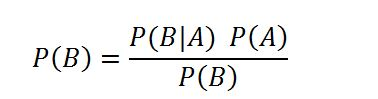Where, A and B are events and P(B) ≠ 0.

Here,

• P(A|B): Conditional probability of occurrence of event A when event B has already occurred.
• P(B|A): Conditional probability of occurrence of event B when event A has already occurred.
• P(A): Probability of occurrence of event A alone without any dependence on other events.
• P(B): Probability of occurrence of event B alone without any dependence on other events.

## Derivation of Bayes Theorem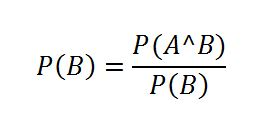Similarly,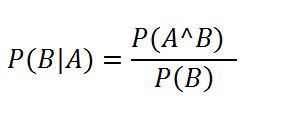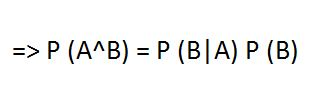Putting the value of P (A^B) in equation (1), we get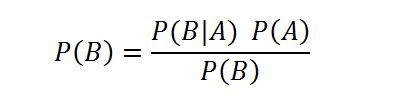Which is our required Bayes equation.

It should be noted that in the Bayesian equation, we need not find the probability of both the events occurring simultaneously, i.e. P(A^B). We can simply calculate the conditional probability of an event if we know the conditional probability of the event on which it is dependent and the individual probabilities of both the events without any dependency on each other.

Bayes Theorem is applicable only in those experiments where we have only two events. It is not applicable to the cases where the number of events is more than two.

## Conclusion

Nowadays, Bayes Theorem is used in many areas, and we can find its applications in various fields. For example, in chemical engineering, Bayes theorem is used to predict the drug concentrations in a body, it is also used to anticipate the viability of generated H2 by the electrocatalyst made for hydrogen evolution process. It is also used to find the estimates that how likely a person is prone to cancer depending upon his/her age. Apart from these examples, the Bayes rule is widely used and this theorem has proved to be a very efficient method to find the conditional probabilities.